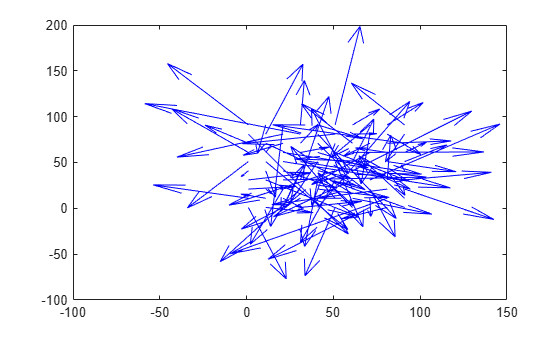# opticalFlow

Object for storing optical flow matrices

## Description

The `opticalFlow` object stores the direction and speed of a moving object from one image or video frame to another. Use the object function `plot` to plot the optical flow vectors.

## Creation

### Syntax

``flow = opticalFlow``
``flow = opticalFlow(Vx,Vy)``

### Description

example

````flow = opticalFlow` creates an optical flow object with default property values. ```

example

````flow = opticalFlow(Vx,Vy)` creates an optical flow object from two equal-sized matrices `Vx` and `Vy`. The matrices `Vx` and `Vy` are the x and y components of velocity respectively. The input velocity components set the Properties of optical flow object.```

### Input Arguments

expand all

x component of velocity, specified as an M-by-N matrix.

Data Types: `single` | `double`

y component of velocity, specified as an M-by-N matrix.

Data Types: `single` | `double`

Note

`Vx` and `Vy` must be of the same size and data type.

## Properties

expand all

x component of velocity, specified as an M-by-N matrix. If the input `Vx` is not specified, the default value of this property is set to 0-by-1 empty matrix.

Data Types: `single` | `double`

y component of velocity, specified as an M-by-N matrix. If the input `Vy` is not specified, the default value of this property is set to 0-by-1 empty matrix.

Data Types: `single` | `double`

Phase angles of optical flow in radians, specified as an M-by-N matrix of the same size and data type as the components of velocity. The phase angles of optical flow is calculated from the x and y components of velocity. If the inputs `Vx` and `Vy` are not specified, the default value of this property is set to 0-by-1 empty matrix.

Data Types: `single` | `double`

Magnitude of optical flow, specified as an M-by-N matrix of the same size and data type as the components of velocity. The magnitude of optical flow is calculated from the x and y components of velocity. If the inputs `Vx` and `Vy` are not specified, the default value of this property is set to 0-by-1 empty matrix.

Data Types: `single` | `double`

## Object Functions

 `plot` Plot optical flow vectors

## Examples

collapse all

Create an optical flow object and view its properties.

`flow = opticalFlow`
```flow = opticalFlow with properties: Vx: [0×1 double] Vy: [0×1 double] Orientation: [0×1 double] Magnitude: [0×1 double]```

The default values of the properties are returned as an empty matrix of size 0-by-1 and data type `double`.

Create an optical flow object from two equal-sized velocity matrices.

```Vx = randn(100,100); Vy = randn(100,100); opflow = opticalFlow(Vx,Vy);```

Inspect the properties of the optical flow object. The orientation and the magnitude are computed from the velocity matrices.

`opflow`
```opflow = opticalFlow with properties: Vx: [100x100 double] Vy: [100x100 double] Orientation: [100x100 double] Magnitude: [100x100 double] ```

Plot the velocity of the object as a quiver plot.

`plot(opflow,'DecimationFactor',[10 10],'ScaleFactor',10);`